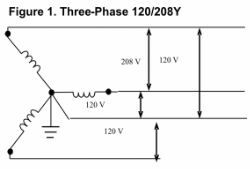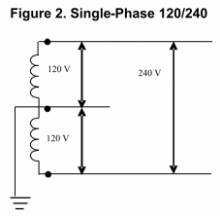DIGITAL PHASE CONVERTERS • THREE-PHASE MOTORS • ROTARY PHASE CONVERTERS • STATIC PHASE CONVERTERS • BUCK/BOOST TRANSFORMERS

 Phase Converters & Power Factor Phase Converter Efficiency Installing a Phase Converter Rotary Phase Converters Static Phase Converters VFDs as Phase Converters      • Harmonic Distortion Three-Phase Motors Phase Converters & Voltage Balance Phase Converter Applications      • Submersible Pumps      • Woodworking Equipment      • Dual Lift Stations      • Phase Converters & Welders      • Phase Converters & CNC Machines      • Phase Converters & Air Compressors      • Phase Converters & Elevators      • Phase Converters & Wire EDM      • Phase Converters & HVAC Phase Converters & Transformers      • Step-up Transformers      • Buck-Boost Transformers      • Isolation Transformers Phase Converter Experts Digital Phase Converters Regenerative Power Three-Phase Power      • Delta vs. Wye Configured Power Motor Starting Currents

# Phase Converters and Delta vs. Wye Configured Three-Phase PowerWeb www.phaseconverterinfo.com

## There are two basic configurations of three-phase power, delta and wye.  An understanding of these two types of power may be important for the safe operation of three-phase equipment.

Wye Three-Phase
Three-phase power is most commonly provided by the electric utility in a wye configuration. The main advantage to wye power is that the phase-to-neutral voltage is equal on all three legs. This is an important consideration because three-phase lines are the backbone of the electrical distribution system, and three-phase service is commonly provided to large buildings and facilities to power both three-phase loads and 120 volt single-phase loads. In North America electrical systems, 120 volt power is almost always derived from a phase to neutral connection. This is true of both single-phase service, which is provided as 120/240 volt, and three-phase service as 120/208Y.

In the above systems, 120 volts is derived from a phase-to-neutral connection in both cases. The 240 volts of the single-phase system and the 208 volts of the three-phase system are both derived as phase-to-phase voltages. In order to have 120 volts from phase to neutral on both systems, the phase-to-phase voltages of the systems must be different. This can best be understood with the help of the phase vector diagrams in figures 1 and 2.Figure 1 represents 120/208V wye, which is delivered with four wires composed of one wire for each voltage and a neutral. Each of the three voltages are 120 degrees apart and have a common connection to ground and neutral (which are at the same potential) represented by the dot in the middle. The voltage measured between any two phases would be 208V, while any phase-to-neutral voltage would be 120V. The relationship between these voltages can be expressed mathematically. Specifically, in a wye configured system, the phase-to-neutral voltage is equal to the phase-to-phase voltage divided by the square root of three, or 208V / 1.73 = 120V. Thus, the phase-to-phase voltage must be 208V in order to get 120V from the system.Figure 2 represents 120/240V single-phase with two voltages 180 degrees apart with neutral and ground halfway between the two voltages.  The voltage measured between the two legs is 240V, and since neutral is halfway between, the voltage from either leg to neutral would be 120V.

In rare instances, three-phase power from the electric utility is configured as 120/240V delta. This is represented in Figure 3.

In this configuration, ground and neutral are not referenced equally to the three legs, but are half-way between the voltage derived from two of the legs.  As you can see, the voltage from leg 1 or leg 2 to neutral would be 120V, but the voltage from the third leg will

be much higher, approximately 208 volts.  With 120/240V delta three-phase, single-phase 120V equipment must never be powered by deriving a phase-to-neutral voltage from the third phase because it will be 208 volts.  Because of this high voltage to ground and neutral, this leg is often referred to as the “high leg”.

##### Delta Configured Three-Phase Power from a Phase Converter

Nearly all phase converters produce three-phase power by generating a voltage that is added to the two legs of single-phase.  The phase vector diagram in Figure 3 helps to illustrate how this is accomplished.In the figure, 1 and 2 represent the two legs of single-phase service which are 180 degrees apart and have a potential of 240 volts between them.  Ground and neutral

are halfway between these two voltages. The phase converter generates voltage 3 at right angles to the voltage between 1 and 2.  If this voltage is generated at the proper level and at the correct angle, it will create a triangle when referenced to voltages 1 and 2 of the single-phase service. In essence, it is the same as the 120/240V delta power sometimes provided by the utility that was discussed earlier. The same warnings apply to deriving a phase-to-neutral voltage from the third phase. While the phase-to-neutral voltage from leg 1 or 2 is 120 volts, leg 3 to neutral (and ground which is at the same potential as neutral) will be approximately 208 volts. This is because in order to have balance phase-to-phase voltages, voltage 3 must be equal to one-half the voltage of leg 1 to leg 2 times the square root of three or, 240V / 2 = 120V x 1.73 = 208V.

If a piece of three-phase equipment operates only on phase-to-phase voltages, it does not matter whether the power is configured as wye or as delta. If none of the circuits in the machine or the motor being operated derive a voltage by referencing neutral/ground, the machine does not know or care where ground/neutral is in reference to any of the phases. It only “sees” the relationship between the phases. It is evident that if you reference the three voltages of wye power and “erase” the connection to ground/neutral, you have a triangle, the same as in delta configured power. Only when equipment has single-phase 120V circuits that are derived from phase-to-neutral connections does it matter if the power is configured as wye or delta.

If the three-phase equipment has 120V phase-to-neutral circuits that cannot be isolated and connected to either leg 1 or leg 2, the output of the phase converter must be passed through a 240V delta to 120/208V wye isolation transformer. This transformer will establish ground and neutral at equal distance from all three legs and deliver the power in a wye configuration.

This is especially important when operating some newer CNC machines that have certain Fanuc or Siemens controls. These controls handle regenerative power from the spindle motor by putting the regenerative power back onto the line utilizing a phase-to-neutral connection on all three legs. If these machines are powered by a phase converter with delta configured power, the regen circuit on the leg manufactured by the phase converter will operate at a voltage that is too high. An isolation transformer must be used to convert the power from delta to wye configuration.